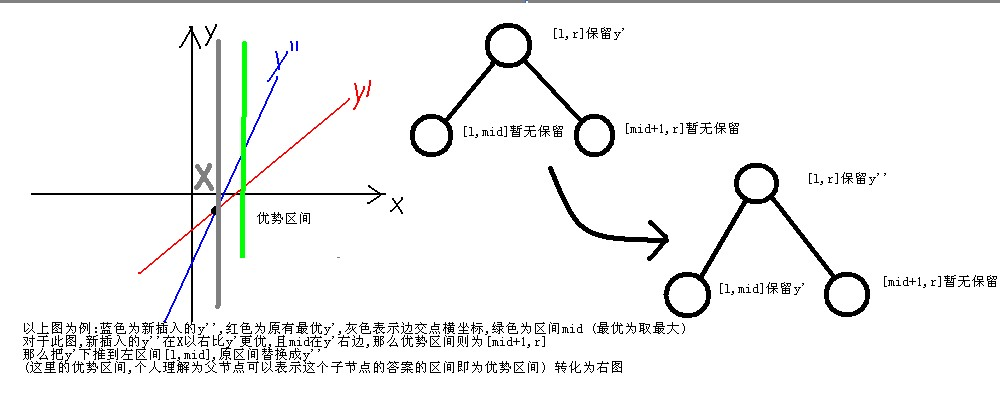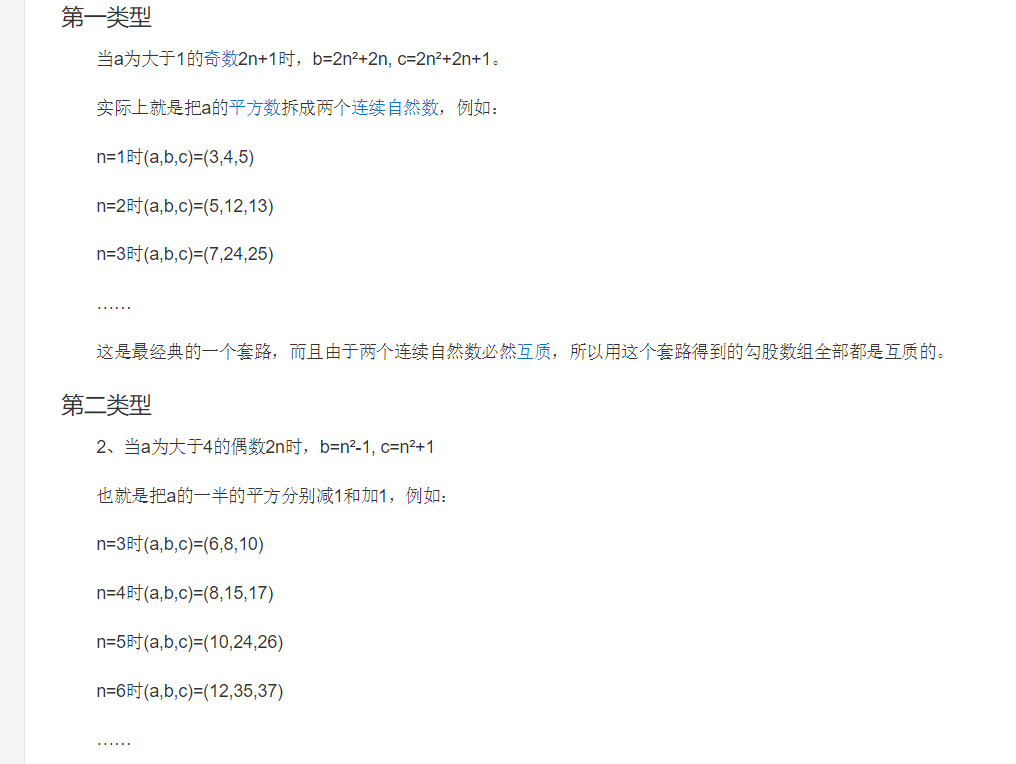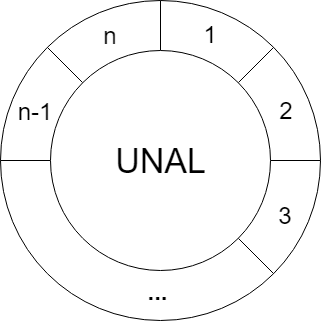# HDU - 1890 Robotic Sort 伸展树

``````#include <iostream>
#include <cstdio>
#include <algorithm>
using namespace std;
const int N = 2e5 + 10;
struct Splaytree {int nex[N]; int f[N];int sz[N];  // 子树节点个数 int val[N]; // 当前节点的值int cnt[N]; // 当前节点个数 int rev[N]; // 翻转标记 int root;int tot;void init() {root = 0;tot = 0;f = sz = nex = nex = 0;rev = 0;cnt = 0;}void newnode(int rt, int v, int fa) {f[rt] = fa;sz[rt] = 1;val[rt] = v;cnt[rt] = 1;rev[rt] = 0;nex[rt] = nex[rt] = 0;} void delnode(int rt) {f[rt] = sz[rt] = val[rt] = cnt[rt] = 0;nex[rt] = nex[rt] = 0;}void pushup(int rt) {if(!rt) return;sz[rt] = cnt[rt];if(nex[rt]) sz[rt] += sz[nex[rt]];if(nex[rt]) sz[rt] += sz[nex[rt]];}void build(int &rt, int l, int r, int fa) { // 构建 if(l > r) return;int mid = (l + r) >> 1;rt = mid;	val[rt] = mid;newnode(rt, val[rt], fa);cnt[rt] = 1;build(nex[rt], l, mid - 1, rt);build(nex[rt], mid + 1, r, rt);pushup(rt);}void update_rev(int rt) {if(rt) {nex[rt] ^= nex[rt] ^= nex[rt] ^= nex[rt];rev[rt] ^= 1;}}void pushdown(int rt) {if(!rt) return;if(rev[rt]) {update_rev(nex[rt]);update_rev(nex[rt]);rev[rt] = 0;}}void rotate(int x, int k) { // 0：左旋 1：右旋  int y = f[x];int z = f[y];pushdown(y);pushdown(x);nex[y][!k] = nex[x][k];if(nex[x][k]) f[nex[x][k]] = y;f[x] = z;if(z) nex[z][nex[z] == y] = x;f[y] = x;nex[x][k] = y;pushup(y);pushup(x);}void splay(int x, int goal) { // x 旋转到 goal下面 while(f[x] != goal) {if(f[f[x]] == goal) rotate(x, nex[f[x]] == x);else {int y = f[x], z = f[y];int K = (nex[z] == y);if(nex[y][K] == x) rotate(x, !K), rotate(x, K);else rotate(y, K), rotate(x, K);}} pushup(x);if(goal == 0) root = x;}int rt_right(int rt){ // 子树 最右 for(pushdown(rt); nex[rt]; pushdown(rt)) rt = nex[rt];return rt;}void delete_rt() { // 删除 根节点 if(nex[root] == 0) {root = nex[root];f[root] = 0;} else {int y = rt_right(nex[root]);splay(y, root);nex[y] = nex[root];f[nex[root]] = y;root = y;f[root] = 0;pushup(root);}}}p;
int n;
struct node {int x, id;bool operator <(const node &b)const {if(x == b.x) return id < b.id;else return x < b.x;}
}a[N];
int main() {while(~scanf("%d", &n) && n) {for(int i = 1; i <= n; i++) {scanf("%d", &a[i].x);a[i].id = i;}sort(a + 1, a + 1 + n);p.init();p.build(p.root, 1, n, 0);p.pushup(p.root);for(int i = 1; i <= n; i++) {p.splay(a[i].id, 0);printf("%d%c", p.sz[p.nex[p.root]] + i, " \n"[i == n]);p.update_rev(p.nex[p.root]);p.delete_rt();}}return 0;
}``````

### 相关文章：## 2019百度之星 - 复赛 HDU-6725 Diversity 树形dp## 2019百度之星 - 复赛 HDU-6726 Transformation 搜索剪枝## 2019百度之星 - 复赛 HDU-6727 Quasi Binary Search Tree 构造 二叉树## 2019 南京网络赛 A.The beautiful values of the palace 构造螺旋矩阵 + CDQ / 离线 + 树状数组## 2019南京网络赛 D.Robots 树上期望dp## bzoj1568: [JSOI2008]Blue Mary开公司 李超线段树## 牛客练习赛51 C、勾股定理 只一边求另外两边 结论## 2019徐州网络赛 G Colorful String 马拉车+后缀## 2019徐州网络赛 I query 线段树 离线## 2019徐州网络赛 J、Random Access Iterator 树上 概率 dp## 2019银川网络赛A？ FZU - 2105 Digits Count 线段树 区间操作 | ^## 2019 徐州网络赛 F. Little M's attack plan 树上倍增+dfs序+二分## HDU - 5489 Removed Interval LIS+枚举技巧## 2019 南昌网络赛 C Hello 2019 / CodeForces - 750ENew Year and Old Subsequence 线段树维护矩阵## 构造螺旋矩阵 n*m## 2019 上海网络赛 B、 Light bulbs 区间差分 / 线段树点维护区间## 2019 上海网络赛 G、Substring 字符串哈希## 2019 沈阳网络赛 H. Texas hold'em Poker 模拟## 2019 沈阳网络赛 B. Dudu's maze dfs 联通块选取## 51nod 1571 最近等对 CQD分治## 牛客 Fruit Ninja 随机大法## HDU - 6242 Geometry Problem 随机数## HDU - 6393 Traffic Network in Numazu 树上倍增求lca+dfs序+线段树## CodeForces - 1220D Alex and Julian 二分图性质## CodeForces - 1220E Tourism 无向图缩点+树形dp## HDU - 6156 Palindrome Function 数位dp## HDU - 6153 A Secret 扩展kmp## Gym - 100589A Queries on the Tree 树状数组+分块## HDU-6731 Angle Beats 计算几何 直角三角形个数## HDU - 5927 Auxiliary Set dfs序+瞎搞## CodeForces - 689D Friends and Subsequences ST表+二分## 2017南宁 I. Rake It In dfs博弈## CodeForces - 369E Valera and Queries 树状数组+离线+容斥思想## Gym - 101889I Imperial roads 树链剖分+最小生成树之必选一边## CodeForces - 1228C Primes and Multiplication 数贡献## CodeForces - 1228D Complete Tripartite 图上的哈希## 2017 ICPC Asia Urumqi I. A Possible Tree 带权并查集## HDU - 5923 Prediction 多个并查集## 2018徐州 Gym - 102028J Carpets Removal 概率事件+二维差分## Gym - 102307J Jail Destruction 线段树## Gym - 101955L Machining Disc Rotors 计算几何 圆剩余部分直径## Gym - 102307E Extreme Image 线段树 扫描线## Gym - 102307C Common Subsequence dp## 18南京 Gym - 101981M Mediocre String Problem 扩展kmp+马拉车## CodeForces - 374D Inna and Sequence 线段树## ICPC Asia HongKong 2017## 1225 D. Power Products 哈希## 1080 F. Katya and Segments Sets 主席树